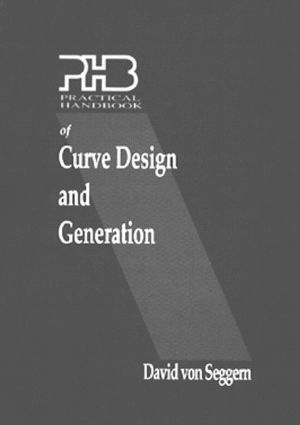# Practical Handbook of Curve Design and Generation

## 1st Edition

CRC Press

288 pages

Hardback: 9780849389160
pub: 1994-03-08
\$250.00
x

FREE Standard Shipping!

### Description

Computers are now being used virtually everywhere in arts, drafting, and design to generate curves and surfaces ranging from the elementary to the intricate. Practical Handbook of Curve Design and Generation is a ready reference that presents the basic mathematics of curves in a complete, clear manner that enables you to apply the material to your own work with minimum effort. By knowing how curves are mathematically generated and how their shape is controlled, you can more fully exploit available computer tools, modify these tools themselves, and provide input for others to modify them. It will also help you to identify mathematical equations required to produce specific curves. The book does not require a heavy mathematical background-if you understand elementary algebra and trigonometry, you can fully apply the material presented. Essential mathematical concepts are repeated in the book to reinforce your knowledge of those topics.

Featuring some 300 graphic examples, the book is organized so that early chapters cover fundamental polynomial, trigonometric, and exponential forms. The mathematical transformation of curves is then treated in order to give you a general approach for modifying known curves. Later chapters introduce complex curves that can be composed from the building blocks presented in earlier chapters. The final chapters cover interesting ideas in space curves and in surfaces.

Introduction to Mathematical Functions. Polynomials. Radicals. Exponential Functions. Periodic Functions on a Line. Periodic Functions on a Circle. Spirals. Non-Periodic Functions on a Line. Closed Curves without Periodicity. Transformation of Curves. Complex Curves from Polynomials. Piecewise Curves. Complex Curves from Harmonics. Complex Curves from Wavelets. Space Curves. Surfaces from Curves.